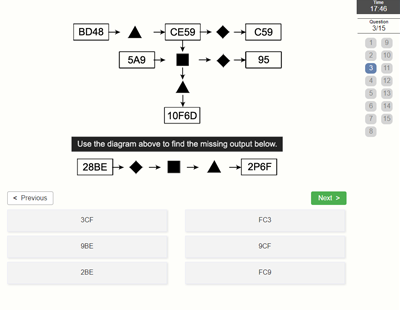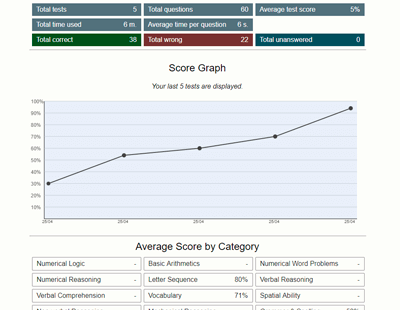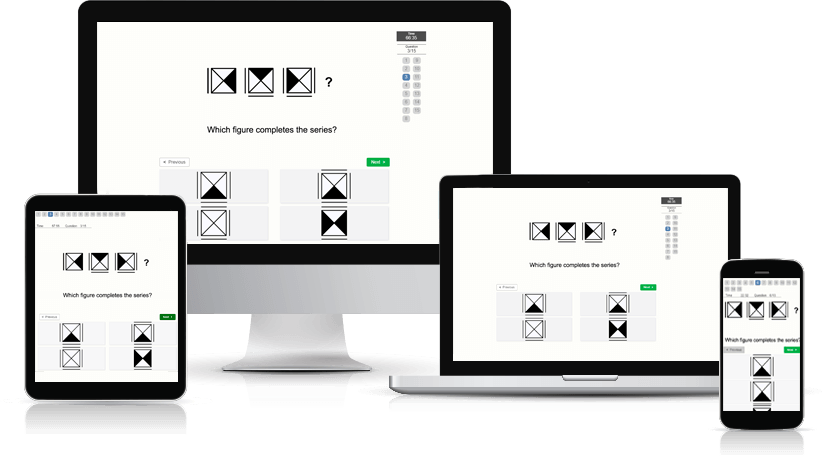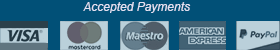﻿ Diagrammatic Reasoning Test - Aptitude-Test.com

# Diagrammatic Reasoning Test

Diagrammatic reasoning tests assess your ability to think logically and analytically and to solve complex problems. The term “diagrammatic reasoning” is sometimes used to describe what we call abstract reasoning questions.

What questions can I expect?
There are several different common types of diagrammatic reasoning questions – we will describe each of these. The names we use below are descriptive names that we have made up and are not standardized terms.

Operators:
This is one of the most common types of diagrammatic reasoning question. You are presented with a list of “operators” and an input diagram. The list of “operators” will each affect the input diagram in specific ways.
You then have to determine how the input diagram will look after being affected by the operations of the icons. In short: You have to find the correct output diagram.

It can be hard to understand without an example. Click here to view an example question.

Input/Output:
In this type of question you also have “operators,” but unlike the Operators question type, you do not know their function beforehand.
You are given a sample diagram that you have to use in order to determine the functions of the “operators.” In this sample diagram there are one or more large input boxes that are processed through one or more small “operator” boxes to result in one or more large output boxes.
You are then given a simple diagram with one large input box and one or more small “operator” boxes with the output box missing. You then have to determine how the output box will look after being processed.

Comparing Sets:
In this type of question you are first presented with two sets of figure series.
You are then given one figure and have to determine which of the two sets, if any, the figure belongs to.

Where are Diagrammatic Reasoning tests used?
Diagrammatic reasoning tests are frequently used for jobs where problem-solving and logical thinking abilities are important, such as software developers and analysts.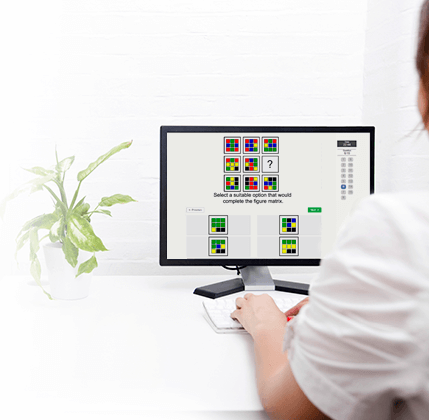## Practice Diagrammatic Reasoning Test

Try a free Diagrammatic Reasoning Test.
This practice test containing 8 test questions with a time limit of 10 minutes.

##### Would you like to improve your test score? Practice smart with a Test Prep Account.

Practice on 100 diagrammatic reasoning questions and a total of 439 non-verbal aptitude questions with detailed description and score statistics.

The many features of a Test Prep Account:

• Preparation software developed under a didactic and methodical perspective
• High-quality practice test questions
• Clearly explained solutions
• Accessible on all devices
• Detailed score data and progression charts
• Reference scores to compare your performance against others
• Training Assistant
• Practice mode (feedback after every question and no time limit)